Function Repository Resource:

# ChainD

Get the derivative with respect to a function

Contributed by: Carl Woll
 ResourceFunction["ChainD"][f, g] gives the partial derivative of f with respect to g, where both f and g are functions of the same variable. ResourceFunction["ChainD"][f, {g, n}] gives the nth derivative. ResourceFunction["ChainD"][f, g1,g2,…] gives the partial derivative of f with respect to g1 followed by the partial derivative with respect to g2, etc.

## Details and Options

Only derivatives with respect to univariate functions are supported.
When differentiating with respect to multiple functions, each function must be a univariate function, but they need not be functions of the same variable.
Derivatives with respect to different functions are, in general, not commutative.
A symbolic inverse of the function g(x) is not required.
Derivatives with respect to functions are obtained using the relation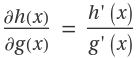.

## Examples

### Basic Examples (2)

Derivative of x4 with respect to x2:

 In:=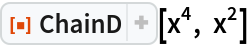Out=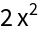Derivative of a more complicated function:

 In:=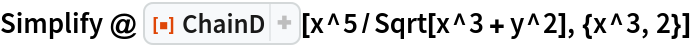Out=### Scope (2)

It is not necessary to be able to symbolically invert g(x):

 In:=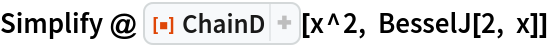Out=It is not possible to symbolically invert BesselJ[2,x]:

 In:=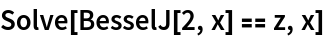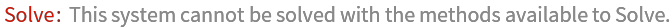Out=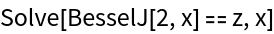Derivative of a multivariate function with respect to functions of each of the variables:

 In:=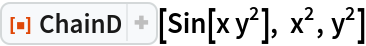Out=### Possible Issues (2)

When using ChainD with multiple functions, the result in general depends on the order of derivatives:

 In:=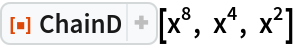Out=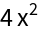In:=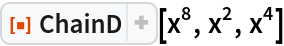Out=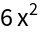Derivatives with respect to heads of functions are not supported:

 In:=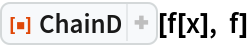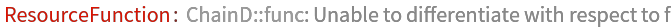Out=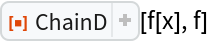Carl Woll

## Version History

• 2.0.0 – 28 February 2020
• 1.0.0 – 23 October 2019